# IBPS Clerk Mains 2017 – Quantitative Aptitude DAY 10

Dear Banking Aspirants,

IBPS CLERK Mains 2017, the last exam of the year 2017 is going to held in 21.01.2018.There is only few days in our hands for preparation and you have to work hard to achieve your goal, because the competition is very high as per the vacancy is concerned.

So start your preparation now with this study Planner for IBPS Clerk Mains. It’s a 20 days complete planner with various Aptitude, Reasoning, English, Current affairs & Static Gk topics Included. There are 4 Mock tests available in this planner.

We have planned this Planner in Such a way that this will cover the entire current affairs quiz from the month of October to January (1st week). Because Banking Awareness, Current Affairs, Static Gk & Computer Awareness will play a vital role in cracking the IBPS Clerk Mains exam. Though the exam is getting tougher and tougher for each and every Year, Only consistently hard working candidates will crack the exam. So be a continuous hard worker and grab the opportunity to achieve your success.

### Make hay while the Sunshine!!!!!!!!!!!

So as per the planner here is content related to Quantitative Aptitude .

IBPS CLERK Mains 2017 -Quantitative Aptitude DAY 10

Quantitative Aptitude

Time: 20 Minutes

Topic: Missing number/problem on train

### Missing number/problem on train

1. 4500, 5400, 5488, 4608, ___?
2. 3, 6, 17, 48, ___, 248
3. 13, 24, 42, 79, 153, ___?
4. 2, 4, 20, 74, ___, 452
5. 122, 131, 203, 446, ___?
6. 6, 1338, 3074, 5298, ___?
7. 2199, 868, 139, 14, ___?
8. 3, 36, 153, 408, ___?
9. 10, 36, 92, 190, 342, ___?
10. 2, 65, 730, 4097, ___?

11) How long will a train 60 m long travelling at 40 Km an hour,take to pass through a Station whose platform is 90 m long?

a)14 SEC

b)13.5 SEC

c) 14.5 SEC

d)15 SE

e)NONE OF THESE

12)Two trains 121 metres and 99 metres in length respectively are running in opposite direction,one at the rate of 40 km  and the other at the rate of 32 km an hour.In what time will they be completely clear of each other from the moment they meet?

a)10 SEC

b)11 SEC

c)12 SEC

d)13 SEC

e)14 SEC

13) A train running at 25km/he takes 18 seconds to pass a platform .Next,it takes 1 Seconds to pass a man waling at 5 km.hr in the opposite direction.Find the length of the train and that of the platform?

a)100 M,150 M

b)125,100 M

c)100,25 M

d)125,150 M

e)NONE OF THESE

14) 150 metre long train crosses a platform of length 250 metre in 30 seconds.Find the time for train to cross a bridge  of 130 metre?

a)21 SECONDS

b)15 SECONDS

c)10 SECONDS

d)25 SECONDS

e)NONE OF THESE

15) 120 metre long train crosses a tunnel of length 80 metres in 20 seconds .Find the time for train to cross a man standing on a platform of length 130 metre?

a)10 SECS

b)12 SECS

c)14 SECS

d)16 SECS

e)NONE OF THESE

16) Two Station A and B are 110 km apart on a straight line.A train starts from A towards at the rate of 40 km/hr.@ hours later another train starts from B and travel towards A at the rate of 50 m/hr.When will the first train meet to the second train?

a)5/3 HRS

b)7/3 HRS

c)2 HRS

d)3 HRS

e)NONE OF THESE

Q.17) A train passes by a stationary man standing on the platform in 7 seconds and passes by the platform completely in 28 seconds.If the length of the platform is 330 metres,what is the length  of the train?

a)110 M

b)150 M

c)180 M

d)200 M

e)220 M

Q.18) Two trains of the same length but with different speeds apass a static pole in 4 seconds and 5 seconds respectively.In what time will they cross each other when they are moving in the opposite direction?

a)40/9 SECONDS

b)30/9 SECONDS

c)60/9 SECONDS

d)70/9 SECONDS

e)NONE OF THESE

Q.19) Two trains of length 100 m and 80 m respectively run on parallel lines of rails.When running in the same direction the faster trains passes the slower train in 18 seconds,but when they are running in opposite direction with the same speeds as earlier,they pass each other in 9 seconds.find the speed of each train?

a)15 M/S AND 5 M/S

b)20 M/S AND 25 M/S

c)10 M/S AND 15 M/S

d)20 M/S AND 10 M/S

e)NONE OF THESE

Q.20) A train crosses 210 metres and 122 metres long bridge in 25 seconds and 17 seconds respectively.Find the length and speed of the train?

a)11 M/SEC

b)15 M/SEC

c)18 M/SEC

d)21 M/SEC

e)NONE OF THESE

1. 4500, 5400, 5488, 4608, ____?

Sol:

4500           5400           5488           4608           2916

53×62           63×52           73×42             83×32          93×22

1. 3, 6, 17, 48, ____, 248

Sol: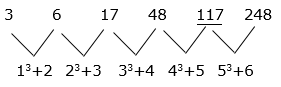3.13. 13, 24, 42, 79, 153, ____?

Sol: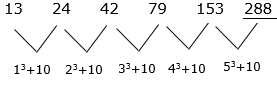1. 2, 4, 20, 74, ____, 452

Sol: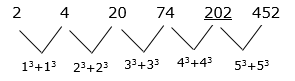1. 122, 131, 203, 446, ____?

Sol: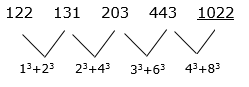1. 6, 1338, 3074, 5298, ____?

Sol: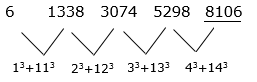7. 2199, 868, 139, 14, ___?

Sol: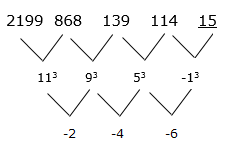8.3, 36, 153, 408, ___?

Sol:

3 = (13 + 13) + 1

408 = (43 + 73) + 1

34 = (23 + 33) + 1

855 = (53 + 93) + 1

153 = (33 + 53) + 1

1. 10, 36, 92, 190, 342, ___?

Sol:

10 = (13+ 23) + 1

190 = (43 + 53) + 1

36= (23 + 33) + 1

342= (53 + 63) + 1

92= (33 + 43) + 1

560 = (63 + 73) + 1

1. 2, 65, 730, 4097, ____?

Sol:

2 = (13 × 13) + 1

4097 = (43 × 43) + 1

65 = (23 × 23) + 1

15626 = (53 × 53) + 1

730 = (33 × 33) + 1

11)Explanation:

Time=distance/Speed

Distance=Length of the Train+Length of the Platform

=60+90=150 m

Speed =40km/hr

Type conversion:

Speed= 40*5/18=11.11

Time=150/11.11

Time=13.5 Seconds

12) Explanation:

They move in Opposite direction therefore Relative Speed =Sum of their speed=40+32 km/hr=72km/hr=20m/sec

Length=121+99=220 metre

Time=Length /Speed=220/20=11 Sec

13) Explanation:

Speed of the train relative to man=25+5=30 km/hr

=30*(5/18)=25/3 m/sec

Distance travelled in 12 seconds at this speed

=25/3 *12=100 m

Speed of the train=25 km/hr=25*(5/18)=125/18 m/sec

Distance travelled in 18 secs at this speed

=125/18*18=125 m

Length of the train + Length of the platform=125 m

Length of the platform=125-100=25 m

14) Explanation:

Speed of the train=150+250/30

=40/3 m/sec

Now,Train has to cover 150+130=280 metre

Required Time=280*3/40 =21 seconds

15) Explanation:

Speed of the train=120+80/20=10 m/sec

Time taken by train to cross the man=120/10=12 sec

16) Explanation:

Let the first train meet the second x hrs after it starts,then

40x+(x-2)*50=110

Distance covered by the first train=40x km

The second train starts 2 hrs after the first starts its journey ,so the distance covered by the second train=50(x-2)

Total distance=110 km

(110+2*50)/(40+50)=210/90=7/3 hours

17) Explanation:

Let the length of the train be x m

Then, Speed of the train=x/7 m per sec

Speed of the train=x+330/28 m per sec

x=7*330/21 =110m

18) Explanation:

Relative Speed when they are moving in the opposite  direction=x/4+x/5=9x/20 m/sec

Required Time=2x/(9x/20)=40/9Seconds

19) Explanation:

Let the speeds of the trains be x m/s and y m/s

When they are moving in the same direction,the relative speed =(x-y)m/s

x-y=100+80/18=10

Similarly, x+y=100+80/9=20

Solving the two equations

x=15 m/s

y=5 m/s

20) Explanation:

Let the length of the train L m and speed of the train be x m/sec

210+L/25=122+L/17=x

L=210*17-122*25/25-17=520/8=65m

Length of the train=65 metres

Speed =210+65/25=275/25=11 m/sec

You can join in our Telegram Channel the invite given below and you can share this with your friends and other aspirants who are preparing for their exams like you.

Caution: You should have Telegram app installed in your mobile or laptop to join the group. If you don’t have telegram app in your mobile. First install the app and click the link given below….